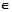# Describe the multiplication modulo p

Publish On: 2019-03-20

Total Post: 542

# Mjay Jollay

Total Post: 0

## ANS: Describe the multiplication modulo p

The multiplication modulo p, p being a positive integer, of any two integers a and b is denoted by a × pb and is defined as

a × pb = r, 0 ≤ r p,

where r is a least non-negative remainder when ab (i.e., the product of a and b) is divided by p. In other words, we find an ordinary product of two integers a and b, viz. ab and from this product we remove the multiples of p in such a way that the remainder r left out is such that either r = 0 or r<p and r is a positive integer.

For example,

8 × 5 7 = 1 as 8 × 7 = 56 = 5.11 + 1

9 × 4 7 = 3 as 9 × 7 = 63 = 15.4 + 3.

Example: Prove that the set {1, 2, 3, 4, 5, 6} is a finite Abelian group of order 6 under multiplication modulo 7.

Solution: Let G = {1, 2, 3, 4, 5, 6} and let us form the composition table X7,

G1: Closure property: From the composition table, it is clear that all the entries are the element of G and, therefore, closure property holds good.

G2: Associative law: For all a, b, cG,

aX7(bX7c) = a × 7(b.c) as b × 7c≡bc (mod 7)

= least non-negative remainder obtained on dividing a(bc) by 7.

= least non-negative remainder obtained on dividing (ab)c by 7.    [∵ (ab)c = a. (bc) ∀ a, b cI]

= (cb) × 7 c

≡ (a × 7b) X7 c     [∵ aX7 b≡ab mod 7]

G3: Identity element: 1G is the identity element as

1 × 7 a = a = aX7 1

G4: Existence of inverse: The inverses of 1, 2, 3, 4, 5, 6 are 1, 4, 5, 2, 3, 6 respectively.

G5: Commutative law: The corresponding rows and columns in the composition table are identical, and as such the commutative law holds good.

Surely, the set G has 6 elements.

Hence <G, × 7> is a finite albelian group of order 6.